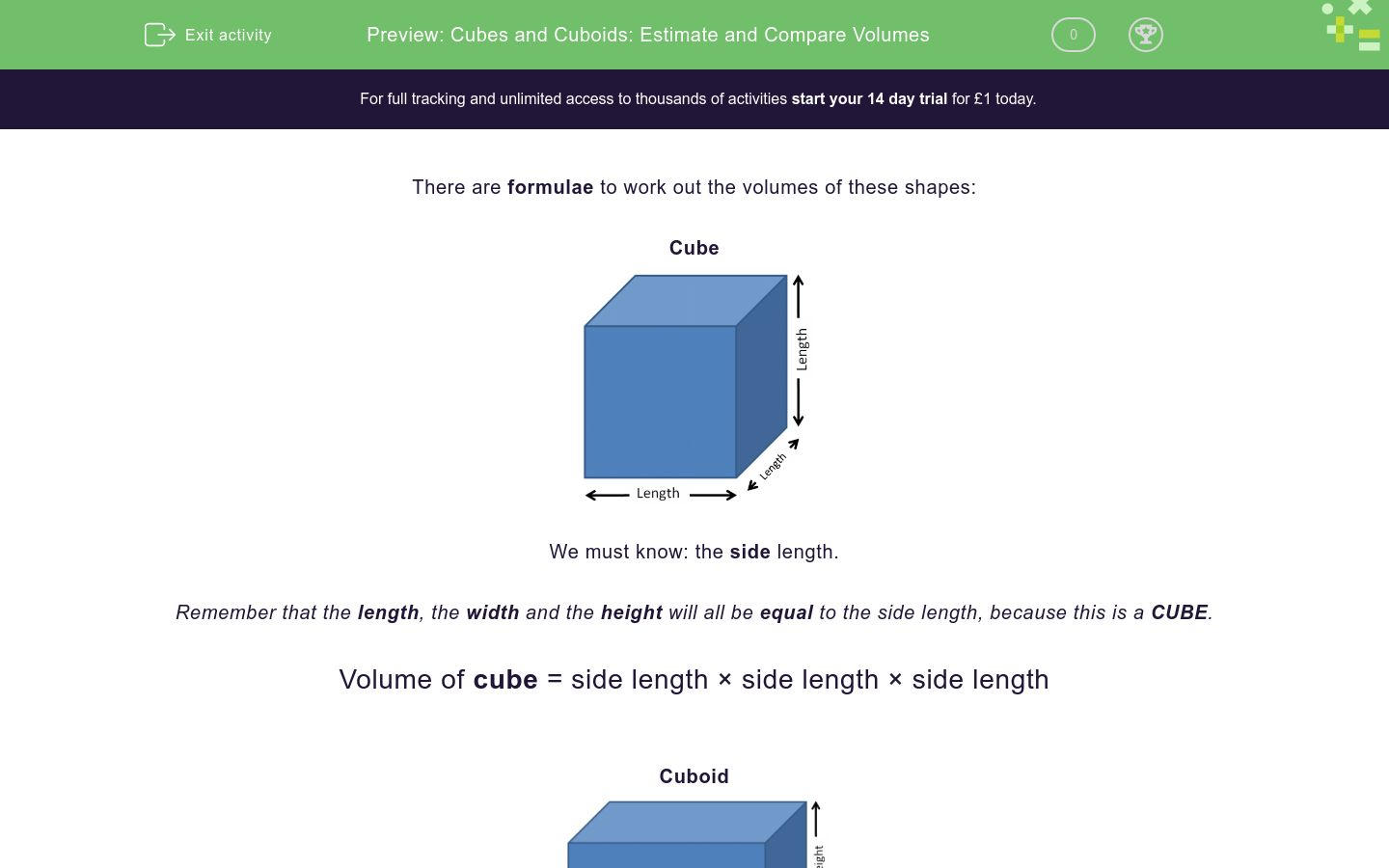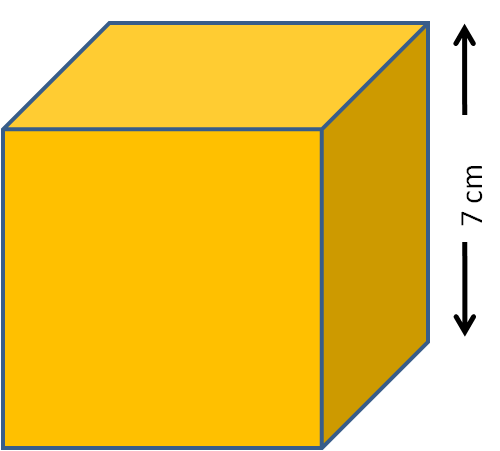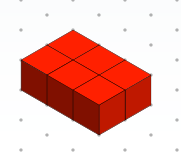# Cubes and Cuboids: Estimate and Compare Volumes

In this worksheet, students must estimate and compare the volumes of the given cubes and cuboids.Key stage:  KS 2

Curriculum topic:   Measurement

Curriculum subtopic:   Use Cubes/Cuboids

Difficulty level:### QUESTION 1 of 10

There are formulae to work out the volumes of these shapes:

CubeWe must know: the side length.

Remember that the length, the width and the height will all be equal to the side length, because this is a CUBE.

Volume of cube = side length × side length × side length

CuboidYou must know: the length; the width; and the height

Volume of cuboid = length × width × height

e.g. How many 1 cm3 cubes will fit into this cuboid?The length is 15 cm, the width is 4 cm and the height is 8 cm.

Volume of cuboid  = length × width × height = 15 × 4 × 8 = 60 × 8 = 480 cm3.

So 480 cubes will fit.

e.g. Calculate the volume of this cube in mm3.The side length is 7 cm, so the width and the height are also 7 cm.

This will be 70 mm.

NB: We want the answer in mm3 which is why we should work in mm not cm.

Volume of cube = side length × side length × side length = 70 × 70 × 70 = 49 × 7 × 10 × 10 × 10 = 343 × 1000 = 343000 mm3.

NB: The length, the width and the height are all interchangeable with each other, depending on how we look at a cuboid.

Want to understand this further and learn how this links to other topics in maths?

Why not watch this video?

How many 1 cm3 cubes will fit into this cuboid?How many 1 cm3 cubes will fit into this cube?Which is larger in volume, the cuboid or the cube?cuboid

cube

both the same

Jack says that the volume of this cube is about 8000 m3.

Is he right or wrong?right

wrong

Which is larger?

The cuboid shown below, or a cube with side length 200 cm?cube

cuboid

both the same

How many 1 m3 cubes will fit into this cuboid?The diagrams show a cuboid and a cube.

How many of the cubes will fit into the cuboid?12

just over 2

just over 3

A large swimming pool is shown below.

Estimate the volume of water in the pool.300 m3

3000 m3

1500 m3

This cuboid is made from 1 cm cubes.

What is the volume of the cuboid in cm3?6 cm3

3 cm3

8 cm3

This cuboid is made from 2 cm cubes.

What is the volume of the cuboid in cm3?6 cm3

12 cm3

48 cm3

• Question 1

How many 1 cm3 cubes will fit into this cuboid?336
EDDIE SAYS
Volume = 12 × 4 × 7 = 336 cm3
• Question 2

How many 1 cm3 cubes will fit into this cube?125
EDDIE SAYS
Volume = 5 × 5 × 5 = 125 cm3
• Question 3

Which is larger in volume, the cuboid or the cube?cuboid
EDDIE SAYS
Volume of cuboid = 5 × 9 × 3 = 135 mm3
Volume of cube = 5 × 5 × 5 = 125 mm3
• Question 4

Jack says that the volume of this cube is about 8000 m3.

Is he right or wrong?wrong
EDDIE SAYS
2 km = 2000 m
Volume = 2000 × 2000 × 2000 which is much bigger than just 8000.
• Question 5

Which is larger?

The cuboid shown below, or a cube with side length 200 cm?cuboid
EDDIE SAYS
Volume of cuboid = 6 × 2 × 1 = 12 m3
Volume of cube = 2 × 2 × 2 = 8 m3
• Question 6

How many 1 m3 cubes will fit into this cuboid?8
EDDIE SAYS
Work in metres.
Volume = 2 × 4 × 1 = 8 m3
• Question 7

The diagrams show a cuboid and a cube.

How many of the cubes will fit into the cuboid?just over 2
EDDIE SAYS
The cube fits into the square end of the cuboid exactly.
2 × 9 = 18 which is nearly 20.
So just over 2 will fit exactly.
• Question 8

A large swimming pool is shown below.

Estimate the volume of water in the pool.1500 m3
EDDIE SAYS
Volume is approximately 30 × 10 × 5 = 300 × 5 = 1500 m3.
• Question 9

This cuboid is made from 1 cm cubes.

What is the volume of the cuboid in cm3?6 cm3
EDDIE SAYS
Each cube has volume 1 cm3.
• Question 10

This cuboid is made from 2 cm cubes.

What is the volume of the cuboid in cm3?48 cm3
EDDIE SAYS
Each cube has volume 2 × 2 × 2 = 8 cm3.
---- OR ----